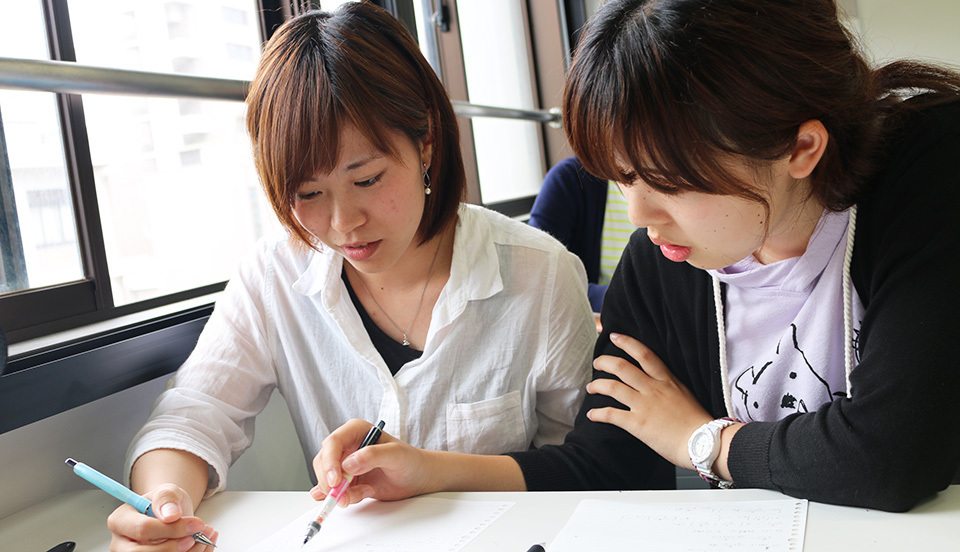The Mathematical Science course offers education and research in mathematics and information science for people who want to study mathematics, or who are interested in computers or information science, or who are aiming to become teachers of mathematics or IT. You will gain broad learning from the basics to applied mathematics, discovering a depth and breadth of mathematics that cannot be experienced at high school. Through the study of mathematics and information science, you will develop the abstract and logical thinking and judgment to be able to solve various problems with flexible concepts that go beneath the surface to the heart of the matter. There are relatively high numbers of female students on the Mathematical Science Course, and we aim to create an environment where women can pursue research equally. The course has three components, Mathematics and Informatics, Applied Mathematics, and Mathematical Analysis. These can be combined to suit your academic and student life.

## Unique

In the mathematical science course, you can study "mathematics" and "information science" in one course. Regarding mathematics, 1st and 2nd grade students will learn the basic contents of algebra, geometry, and analysis based on linear algebra and differential calculus, which are learned in liberal arts education. As the grade goes up, you will study fields related to applications such as differential equation theory, complex function theory, and probability theory. Regarding information science, in the 1st and 2nd grades, students learn computer architecture, computer programming 1, computer mathematics, etc. based on the introduction to information science learned in liberal arts education, and as the grade goes up, numerical computation,  optimization theory, computer graphics basic theory, etc. Learn applied fields. These curriculums can be selected and studied for each individual, focusing on mathematics or informatics.

## Introduction

### Mathematics and Computer Sciences

The Mathematical Science course offers education and research in mathematics and information science for people who want to study mathematics, or who are interested in computers or information science, or who are aiming to become teachers of mathematics or IT. You will gain broad learning from the basics to applied mathematics, discovering a depth and breadth of mathematics that cannot be experienced at high school. Through the study of mathematics and information science, you will develop the abstract and logical thinking and judgment to be able to solve various problems with flexible concepts that go beneath the surface to the heart of the matter. There are relatively high numbers of female students on the Mathematical Science Course, and we aim to create an environment where women can pursue research equally. The course has three components,

### Applied Mathematical Sciences

We offer specialist education in fields from basic to applied mathematics. Class subjects include complex analysis, functional equations, basic mathematics, linear algebra, modeling theory, and applied mathematics.
Graduation research themes include the principles of ghost leg lotteries, the impact of humans on ecosystems, the problem of initial values for equations of heat conduction in n-dimensional space, numerical simulation of flows, the problem of stable marriage considering the unreliability of preference, and portfolio evaluation bearing in mind shareholder preferential treatment systems.

### Mathematical Methods in Sciences

This course offers a broad curriculum of mathematics education for students from the second to the fourth years of science and engineering subjects. Lectures and seminars are used to teach the basic subjects of mathematics relevant to the Department of Science and Engineering overall with a focus on common curriculum subjects. This develops your basic ability in science and engineering, and increases your understanding of professional education. Class subjects include differential equations, vector analysis, complex function theory, and the differential equation advanced course.